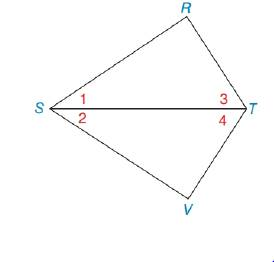Chapter 3.2, Problem 12E### Elementary Geometry for College St...

6th Edition
Daniel C. Alexander + 1 other
ISBN: 9781285195698

#### Solutions

Chapter
Section### Elementary Geometry for College St...

6th Edition
Daniel C. Alexander + 1 other
ISBN: 9781285195698
Textbook Problem
1 views

# In Exercise 5 to 12, plan and write the two-column proof for each problem. Given: ∠ R and ∠ V are right ∠ s R T ¯ ≅ V T ¯Prove: Δ R S T ≅ Δ V S T

To determine

To prove:

The given statement.

Explanation

Given:

The following figure shows the given diagram.

Given that,

R and V are right s and,

RT¯VT¯.

Approach:

By definition of hypotenuse leg congruency (HL), if two triangles have corresponding sides that are then triangle will be congruent.

The completed proof is shown in the following table.

 Proof Statements Reasons 1. ∠R and ∠V are right ∠s 1. Given 2. RT¯≅VT¯ 2. Given 3. ST¯≅ST¯ 3

### Still sussing out bartleby?

Check out a sample textbook solution.

See a sample solution

#### The Solution to Your Study Problems

Bartleby provides explanations to thousands of textbook problems written by our experts, many with advanced degrees!

Get Started

#### In Exercises 2340, find the indicated limit. 32. limx2(x2+1)(x24)

Applied Calculus for the Managerial, Life, and Social Sciences: A Brief Approach

#### In Problems 19-44, factor completely. 20.

Mathematical Applications for the Management, Life, and Social Sciences

#### Rational Inequalities Solve the inequality. 99. 1x2+2x+33x

Precalculus: Mathematics for Calculus (Standalone Book)

#### True or False: is a convergent series.

Study Guide for Stewart's Multivariable Calculus, 8th

#### Proof prove that abx2dx=b3a33.

Calculus: Early Transcendental Functions (MindTap Course List)